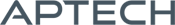# Linear Programming MT

Linear Programming MT

Availability: Out of stock

LPMT is designed to solve small and large scale linear programming problems.
Overview

## Linear Programming MT

Linear Programming MT is designed to solve small and large scale linear programming problems. LPMT exploits sparse matrices and thread-safe execution to tackle even large scale constraint problems with ease. User specified starting values can be initialized to further streamline problems and reduce the number of iterations. ​

Platform: Windows, Mac and Linux.

Requirements: GAUSS/GAUSS Engine/GAUSS Light v8.0 or higher.

Features

Linear Programming MT Module solves the standard linear programming problem with the following features:

• Thread-safe Execution: Control variables are model matrices are contained in structures allowing thread-safe execution of programs.
• Sparse matrices: Linear Programming MT exploits sparse matrix technology permitting the analysis of problems with very large constraint matrices. The size of a problem that can be analyzed is dependent on the speed and amount of memory on the computer, but problems with two to three thousand constraints and more than six thousand variables have been tested on ordinary PC's.
• MPS files: procedures are available for translating MPS formatted files.

Other Product Features

LPMT is designed to solve small and large scale linear programming problems. LPMT can be initialized with a starting value, such as the solution to a previous problem which is similar to the one being solved. This feature can dramatically reduce the number of iterations required to find a feasible starting point.

Features

• Upper and lower finite bounds can be provided for variables and constraints
• Problem type (minimization or maximization)
• Constraint types (<=, >=, =)
• Choice of tolerances
• Pivoting rules
• Sparse matrices
• Compatible with MPS files

Computes

• The value of the variables and the objective function upon termination, and returns the dual variables
• State of each constraint
• Uniqueness and quality of solution
• Multiple optimal solutions if they exist
• Number of iterations required
• A final basis
• Can generate iterations log and/or final report, if requested

Product Inquiry

4 + 7 = enter the result ex:(3 + 2 = 5)

﻿